### Background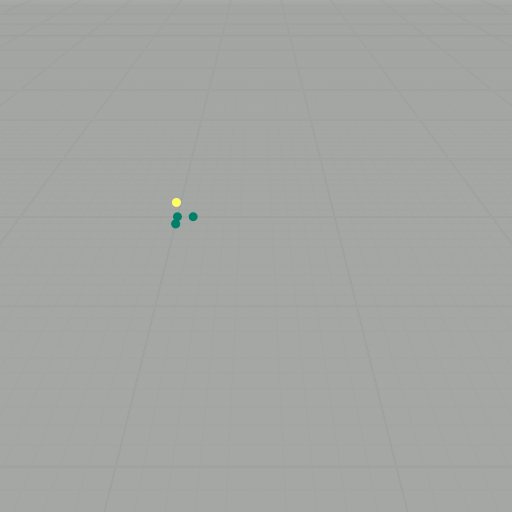Progressive dilations of $$P_n(S)$$ in $$(\mathbb{Z}^3,+)$$

In the commutative setting, vector addition over $$\mathbb{Z}$$ is straightforward. Given two vectors $$v,w \in \mathbb{Z}^3$$, vector addition is simply defined as addition component-wise. In fact, $$(\mathbb{Z}^3, +)$$ actually forms an abelian group, i.e. $$v + w = w + v$$. So now consider some basis set of vectors, say $S = \{ (0,0,0), (1,0,0), (0,1,0), (0,0,1) \}.$ As an example, we will generate all words of length $$k$$. To find these, first take the $$k$$-fold product $$\underbrace{S \times S \times \ldots \times S}_{k \text{ times}}$$. Then for each element in $$S \times S \times \ldots \times S$$, compute $$s_1 \star s_2 \star \ldots \star s_k$$. This will give an element in $$(\mathbb{Z}^3, \star)$$. For $$k = 2$$, this yields \begin{align} (0,0,0) + (0,0,0) & = (0,0,0), \\ (0,0,0) + (1,0,0) & = (1,0,0), \\ (0,0,0) + (0,1,0) & = (0,1,0), \\ (0,0,0) + (0,0,1) & = (0,0,1), \\ (1,0,0) + (0,0,0) & = (1,0,0), \\ (1,0,0) + (1,0,0) & = (2,0,0), \\ (1,0,0) + (0,1,0) & = (1,1,0), \\ (1,0,0) + (0,0,1) & = (1,0,1), \\ (0,1,0) + (0,0,0) & = (0,1,0), \\ (0,1,0) + (1,0,0) & = (1,1,0), \\ (0,1,0) + (0,1,0) & = (0,2,0), \\ (0,1,0) + (0,0,1) & = (0,1,1), \\ (0,0,1) + (0,0,0) & = (0,0,1), \\ (0,0,1) + (1,0,0) & = (1,0,1), \\ (0,0,1) + (0,1,0) & = (0,1,1), \\ (0,0,1) + (0,0,1) & = (0,0,2). \end{align} Getting rid of duplicates, this yields \begin{align} P_2(S) = \{ & (0,0,0) \\ & (0,0,1) \\ & (0,0,2) \\ & (0,1,0) \\ & (0,1,1) \\ & (0,2,0) \\ & (1,0,0) \\ & (1,0,1) \\ & (1,1,0) \\ & (2,0,0) \}, \end{align} where $$P_k(S)$$ denotes the polytope generated as the $$k$$th dilate of the basis set $$S$$. In other words, $$P_k(S)$$ is the set of points realized as words of length $$k$$ generated by elements of the basis set $$S$$. If we let $$\mathcal{B}$$ be an aribitrary (finite) basis set with $$\lvert \mathcal{B} \rvert = n$$ and compute the words of length $$k$$, then there are $$n(n-1)\ldots(n-k+1)$$ evaluations to compute. In this example, the number of points contained in $$P_k(S)$$, which we will call the volume, is given by $$V = \binom{k+2}{3}$$. Furthermore, it can be seen that there are no "missing spots" in the $$P_k(S)$$, so we will say $$P_k(S)$$ is saturated if this is the case. Additionally, the outer face of $$P_k(S)$$ can be desribed by the function $$z = f(x,y) = k-x-y$$. These properties of $$P_k(S)$$ motivate the study of polytopes generated in the setting of the Heisenberg group.

### Setup

To get to the Heisenberg group, we basically do normal vector addition, but now with a twist: $\star \colon \mathbb{Z} \times \mathbb{Z} \longrightarrow \mathbb{Z}, \quad (x, y, z) \star (x', y', z') = (x+x', y+y', z+z'+xy').$ This new operation is almost normal vector addition, except now there is an extra $$xy'$$ tacked onto the $$z$$-component. Because of this new twist, $$(\mathbb{Z}^3, \star)$$ forms a noncommutative group. We want to explore this group some more, so now we will consider the we will choose a basis set of vectors, say $$S$$ (as we did before). As an example, consider again $$k=2$$: \begin{align} (0,0,0) \star (0,0,0) & = (0,0,0), \\ (0,0,0) \star (1,0,0) & = (1,0,0), \\ (0,0,0) \star (0,1,0) & = (0,1,0), \\ (0,0,0) \star (0,0,1) & = (0,0,1), \\ (1,0,0) \star (0,0,0) & = (1,0,0), \\ (1,0,0) \star (1,0,0) & = (2,0,0), \\ (1,0,0) \star (0,1,0) & = (1,1,1), \\ (1,0,0) \star (0,0,1) & = (1,0,1), \\ (0,1,0) \star (0,0,0) & = (0,1,0), \\ (0,1,0) \star (1,0,0) & = (1,1,0), \\ (0,1,0) \star (0,1,0) & = (0,2,0), \\ (0,1,0) \star (0,0,1) & = (0,1,1), \\ (0,0,1) \star (0,0,0) & = (0,0,1), \\ (0,0,1) \star (1,0,0) & = (1,0,1), \\ (0,0,1) \star (0,1,0) & = (0,1,1), \\ (0,0,1) \star (0,0,1) & = (0,0,2). \end{align} Getting rid of duplicates, this yields \begin{align} P_2(S) = \{ & (0,0,0) \\ & (0,0,1) \\ & (0,0,2) \\ & (0,1,0) \\ & (0,1,1) \\ & (0,2,0) \\ & (1,0,0) \\ & (1,0,1) \\ & (1,1,0) \\ & (1,1,1) \\ & (2,0,0) \}, \end{align} Since $$(\mathbb{Z}^3, \star)$$ is noncommutative, it is important that each word is evaluated according to the order of the elements. We can also visualize these results as points in $$\mathbb{Z}^3$$, as shown in the slideshow below. If we let $$\mathcal{B}$$ be an aribitrary (finite) basis set with $$\lvert \mathcal{B} \rvert = n$$ and compute the words of length $$k$$, then there are $$n^k$$ evaluations to compute. For large $$k$$, this task becomes intractable.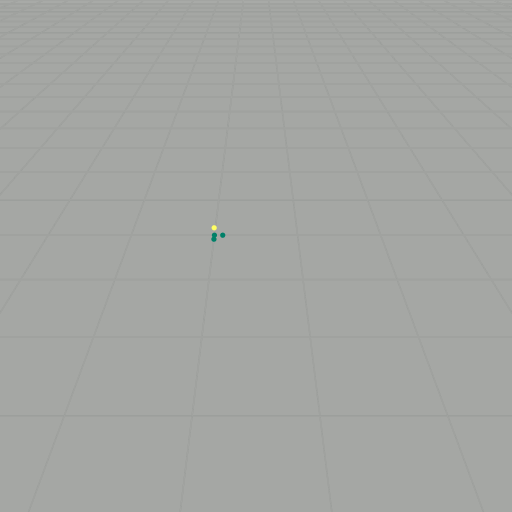Progressive dilations of $$P_n(S)$$ in $$(\mathbb{Z}^3, \star)$$$$P_2(S)$$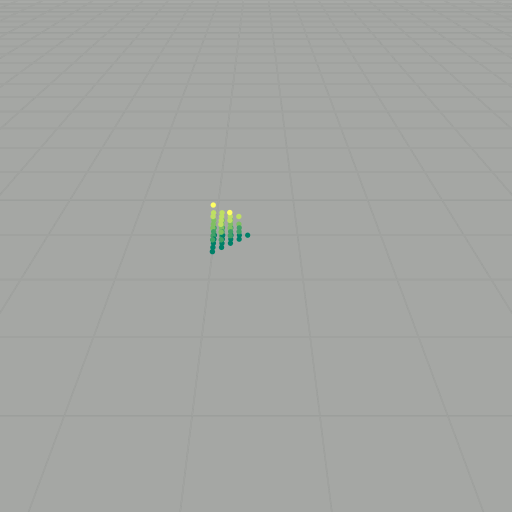$$P_4(S)$$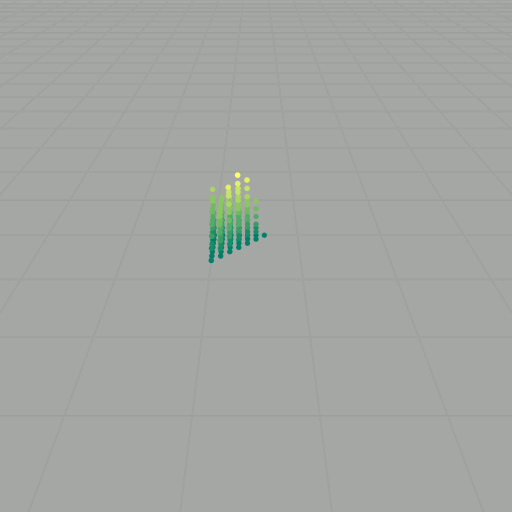$$P_6(S)$$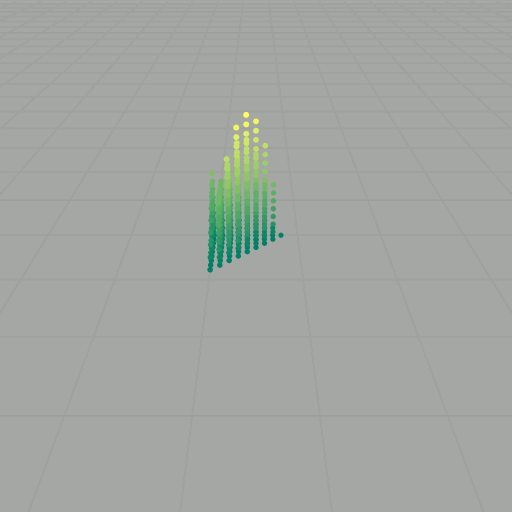$$P_8(S)$$$$P_{10}(S)$$

### Results

We can ask the same questions about $$P_k(S)$$ as we did in the commutative setting:

• What is the volume of $$P_k(S)$$?
• Is $$P_k(S)$$ saturated?
• Can we describe the ceiling function of $$P_k(S)$$?
For this example, since all of the points in $$P-k(S)$$ lie in the positive orthant, if we can find a ceiling function of $$P_k(S)$$, we can also find the volume by integrating. It turns out that the ceiling function for $$P_k(S)$$ is $$z = xy - x - y + k$$. So then by integrating with respect to $$x$$ and $$y$$, we can see that \begin{align*} \lvert P_k(S) \rvert & =\int_{0}^{k} \int_{0}^{k-x} xy - x - y + k \ dy \ dx \\ & =\int_{0}^{k} \int_{0}^{k-x} xy \ dy\ dx + \int_{0}^{k} \int_{0}^{k-x} k - x - y \ dy \ dx \\ & = \int_{0}^{k} \frac{x (k - x)^2}{2} \ dx + \int_{0}^{k} \frac{(k - x)^2}{2} \ dx \\ & = \frac{1}{24} k^4 + \frac{1}{6} k^3 \\ & = \frac{k^4}{4!} + \frac{k^3}{3!}. \end{align*}